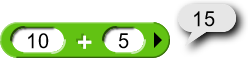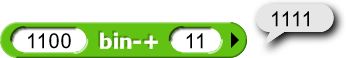# BF in Snap!, Snap!F*** (HELPPPPPP)

Snap! Snap!F*** (berkeley.edu)

How it SHOULD work:
Takes the input, < = 0 and > = 1. It takes your <>'s and turns them into their number (this part works) but then it is supposed to turn them into text by looking at a list. (i need help here < <)

Hello World in Snap!F***

<>><><<< <>><<><> <>><>><< <>><>><< <>><>>>> <<><<<<< <>>><>>> <>><>>>> <>>><<>< <>><>><< <>><<><<


Output:

Hello world


So those numbers are eight-bit ASCII codes? Convert them to numbers and then use UNICODE AS LETTER.

its binary, will encode still work? (im un smart if binary and acsii are the same)

Oh.
My.
GOD.

No, but ASCII codes are in binary. And it looks like you are using ASCII codes there, so I think all you need is a binary-to-decimal thing. I'll go make that.

Edit: There:
Here: Snap! Build Your Own Blocks
Or copy this script pic:

Edit 2: Or check the alternative in the project, which uses two blocks but I think is more efficient.

This pushes one of my buttons. You're not converting the binary (a character string) to decimal (a different character string); you're converting it to a number! Note that, although you operate on the input character by character (with ALL BUT FIRST OF and LETTER LAST OF), you compute the output by doing arithmetic, + and ×. (And note also that there's no mention of 10, ten, as there would be if you were converting to decimal.

It so happens that Snap! converts numbers to decimal numerals when needed for display purposes.

Also, that join of reverse of butfirst of split for each bit isn't very efficient or elegant. How about this:

We really should invent an optional input feature so you could have an input named bits whose default value would be reverse of split of bin, and in the recursive call you could provide that input explicitly with butfirst of bits as its value. Someday...

Should I just use encode decode?

Well you do have to convert the string of bits into a number. Then you can use UNICODE _ AS LETTER.

Already done, I’ll work on it tomorrow.

Is there a reasonshould be a number butnot?

I'd have to look at your code to be sure, but I think the inputs to your bin-+ procedure are numerals (text strings made of digits), not numbers (atomic numeric values with no specific representation).

Snap! goes out of its way to confuse this distinction by automatically converting strings of digits to numbers when you do arithmetic on them, and converting numbers to strings of digits when you do text operations (e.g., JOIN) on them. This automatic conversion is a great help to beginners because they don't have to think about data types at all — until they want to convert to/from binary. And armies of teachers, including the ones who make the code.org videos, exacerbate the situation by pretending there is such a thing as a "binary number." (Numbers have no base; what they mean is "binary numeral.")

This is why your conversion procedure doesn't "convert binary to decimal." It converts binary (numerals) to numbers.

Binary conversion is notoriously hard for students. One reason is that far too many kids get out of elementary school not really understanding place value. But another reason is that they think they're converting numbers to different numbers, and that makes no sense, so it can't lead to inventing an algorithm.

P.S. Those input slots should really be of type Text (or Any), not type Number. This would quickly become obvious if you were dealing with base 16 instead of base 2.

I just used an example, hardcoding the output 1111.

I agree that numbers don't have a base.

I think "number" is an abstract noun--you can't see one. Which is why, in the example I gave there, I believe both outputs to be sequences of numerals, as you can see them.

So which should I do lol.

Yes, I agree, technically even the representation inside the computer isn't a number; it's a (binary) numeral. But the computer's circuitry can do arithmetic on those internal bit sequences, whereas it can't do arithmetic on strings of Unicode characters, even if those characters are all '0' or '1'. So if your goal is to write code, or understand someone else's code, it's useful to make the distinction between strings of Unicode digits ("numerals") and the internal strings of bits ("numbers").

And, in particular, if you're trying to write a function whose domain is "numerals" and whose range is "numbers," or the other way around, I think it's very helpful to keep that distinction in mind. Your code has to do text operations (JOIN, SPLIT, LETTER _ OF, etc.) on "numerals" but arithmetic operations on "numbers."

Snap!'s + function doesn't really operate on strings of decimal digits, even though it looks that way to the user (because, as you say, we can't show the user a number, or even a "number"). What really happens is that Snap! translates the numerals you type into "numbers," the things that computers can do arithmetic on, then adds the two results of that conversion, and then converts back to an external numeral.

Back in the day, Lisp interpreters had two special variables named IBASE (input base) and OBASE (output base) that you could set to change how it did this behind-the-scenes conversion from numerals to "numbers" (IBASE) and from "numbers" to numerals (OBASE). You could even (SETQ OBASE 'ROMAN) and then 3+5 would show its result as VIII. :~)

With the way characters are stored, it technically can. Higher-level languages don't usually permit that, though. (one I can think of that can is Befunge).

Yeah.

Of course it's way more complicated (a half-adder takes two bits and returns two bits (i1 & i2 and i1 ^ i2) and a full adder takes three bits and returns two ((ic & (i1 ^ i2)) | (i1 & i2) and i1 ^ i2 ^ ic)) but...

Interesting. Do you know of any that still work?

Eh? It can, I suppose, have circuitry to convert between digit strings and numbers, but if it just tries to do arithmetic on the digit strings as is, carrying won't work; it'll be as if you were computing in base 256.

Not that modern computers use ripple carry, which takes time proportional to the width of the processor. :~)

I think you can probably find running versions of MacLisp online. I have no idea where my Common Lisp manual is, but if it doesn't do that, I'm sure someone has written an add-on to do it!

Yeah...do you know what most modern computers use?

I looked, and haven't found one yet.

Not exactly. I think it involves a lot of table lookup.